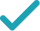Type a math problemSolve for x, ySteps Using Substitution
Steps Using Matrices
Steps Using Elimination
Steps Using Substitution
To solve a pair of equations using substitution, first solve one of the equations for one of the variables. Then substitute the result for that variable in the other equation.
Choose one of the equations and solve it for by isolating on the left hand side of the equal sign.
Subtract from both sides of the equation.
Divide both sides by .
Multiply times .
Substitute for in the other equation, .
Multiply times .
Subtract from both sides of the equation.
Divide both sides of the equation by , which is the same as multiplying both sides by the reciprocal of the fraction.
Substitute for in . Because the resulting equation contains only one variable, you can solve for directly.
Multiply times by multiplying numerator times numerator and denominator times denominator. Then reduce the fraction to lowest terms if possible.
Add to by finding a common denominator and adding the numerators. Then reduce the fraction to lowest terms if possible.
The system is now solved.Giving is as easy as 1, 2, 3
Get 1,000 points to donate to a school of your choice when you join Give With Bing
8x+2y=46,7x+3y=47
To solve a pair of equations using substitution, first solve one of the equations for one of the variables. Then substitute the result for that variable in the other equation.
8x+2y=46
Choose one of the equations and solve it for x by isolating x on the left hand side of the equal sign.
8x=-2y+46
Subtract 2y from both sides of the equation.
x=\frac{1}{8}\left(-2y+46\right)
Divide both sides by 8.
x=-\frac{1}{4}y+\frac{23}{4}
Multiply \frac{1}{8}=0.125 times -2y+46.
7\left(-\frac{1}{4}y+\frac{23}{4}\right)+3y=47
Substitute \frac{-y+23}{4} for x in the other equation, 7x+3y=47.
-\frac{7}{4}y+\frac{161}{4}+3y=47
Multiply 7 times \frac{-y+23}{4}.
\frac{5}{4}y+\frac{161}{4}=47
\frac{5}{4}y=\frac{27}{4}
Subtract \frac{161}{4}=40.25 from both sides of the equation.
y=\frac{27}{5}
Divide both sides of the equation by \frac{5}{4}=1.25, which is the same as multiplying both sides by the reciprocal of the fraction.
x=-\frac{1}{4}\times \left(\frac{27}{5}\right)+\frac{23}{4}
Substitute \frac{27}{5}=5.4 for y in x=-\frac{1}{4}y+\frac{23}{4}. Because the resulting equation contains only one variable, you can solve for x directly.
x=-\frac{27}{20}+\frac{23}{4}
Multiply -\frac{1}{4}=-0.25 times \frac{27}{5}=5.4 by multiplying numerator times numerator and denominator times denominator. Then reduce the fraction to lowest terms if possible.
x=\frac{22}{5}
Add \frac{23}{4}=5.75 to -\frac{27}{20}=-1.35 by finding a common denominator and adding the numerators. Then reduce the fraction to lowest terms if possible.
x=\frac{22}{5},y=\frac{27}{5}
The system is now solved.
8x+2y=46,7x+3y=47
Put the equations in standard form and then use matrices to solve the system of equations.
\left(\begin{matrix}8&2\\7&3\end{matrix}\right)\left(\begin{matrix}x\\y\end{matrix}\right)=\left(\begin{matrix}46\\47\end{matrix}\right)
Write the equations in matrix form.
inverse(\left(\begin{matrix}8&2\\7&3\end{matrix}\right))\left(\begin{matrix}8&2\\7&3\end{matrix}\right)\left(\begin{matrix}x\\y\end{matrix}\right)=inverse(\left(\begin{matrix}8&2\\7&3\end{matrix}\right))\left(\begin{matrix}46\\47\end{matrix}\right)
Left multiply the equation by the inverse matrix of \left(\begin{matrix}8&2\\7&3\end{matrix}\right).
\left(\begin{matrix}1&0\\0&1\end{matrix}\right)\left(\begin{matrix}x\\y\end{matrix}\right)=inverse(\left(\begin{matrix}8&2\\7&3\end{matrix}\right))\left(\begin{matrix}46\\47\end{matrix}\right)
The product of a matrix and its inverse is the identity matrix.
\left(\begin{matrix}x\\y\end{matrix}\right)=inverse(\left(\begin{matrix}8&2\\7&3\end{matrix}\right))\left(\begin{matrix}46\\47\end{matrix}\right)
Multiply the matrices on the left hand side of the equal sign.
\left(\begin{matrix}x\\y\end{matrix}\right)=\left(\begin{matrix}\frac{3}{8\times 3-2\times 7}&-\frac{2}{8\times 3-2\times 7}\\-\frac{7}{8\times 3-2\times 7}&\frac{8}{8\times 3-2\times 7}\end{matrix}\right)\left(\begin{matrix}46\\47\end{matrix}\right)
For the 2\times 2 matrix \left(\begin{matrix}a&b\\c&d\end{matrix}\right), the inverse matrix is \left(\begin{matrix}\frac{d}{ad-bc}&\frac{-b}{ad-bc}\\\frac{-c}{ad-bc}&\frac{a}{ad-bc}\end{matrix}\right), so the matrix equation can be rewritten as a matrix multiplication problem.
\left(\begin{matrix}x\\y\end{matrix}\right)=\left(\begin{matrix}\frac{3}{10}&-\frac{1}{5}\\-\frac{7}{10}&\frac{4}{5}\end{matrix}\right)\left(\begin{matrix}46\\47\end{matrix}\right)
Do the arithmetic.
\left(\begin{matrix}x\\y\end{matrix}\right)=\left(\begin{matrix}\frac{3}{10}\times 46-\frac{1}{5}\times 47\\-\frac{7}{10}\times 46+\frac{4}{5}\times 47\end{matrix}\right)
Multiply the matrices.
\left(\begin{matrix}x\\y\end{matrix}\right)=\left(\begin{matrix}\frac{22}{5}\\\frac{27}{5}\end{matrix}\right)
Do the arithmetic.
x=\frac{22}{5},y=\frac{27}{5}
Extract the matrix elements x and y.
8x+2y=46,7x+3y=47
In order to solve by elimination, coefficients of one of the variables must be the same in both equations so that the variable will cancel out when one equation is subtracted from the other.
7\times 8x+7\times 2y=7\times 46,8\times 7x+8\times 3y=8\times 47
To make 8x and 7x equal, multiply all terms on each side of the first equation by 7 and all terms on each side of the second by 8.
56x+14y=322,56x+24y=376
Simplify.
56x-56x+14y-24y=322-376
Subtract 56x+24y=376 from 56x+14y=322 by subtracting like terms on each side of the equal sign.
14y-24y=322-376
Add 56x to -56x. Terms 56x and -56x cancel out, leaving an equation with only one variable that can be solved.
-10y=322-376
-10y=-54